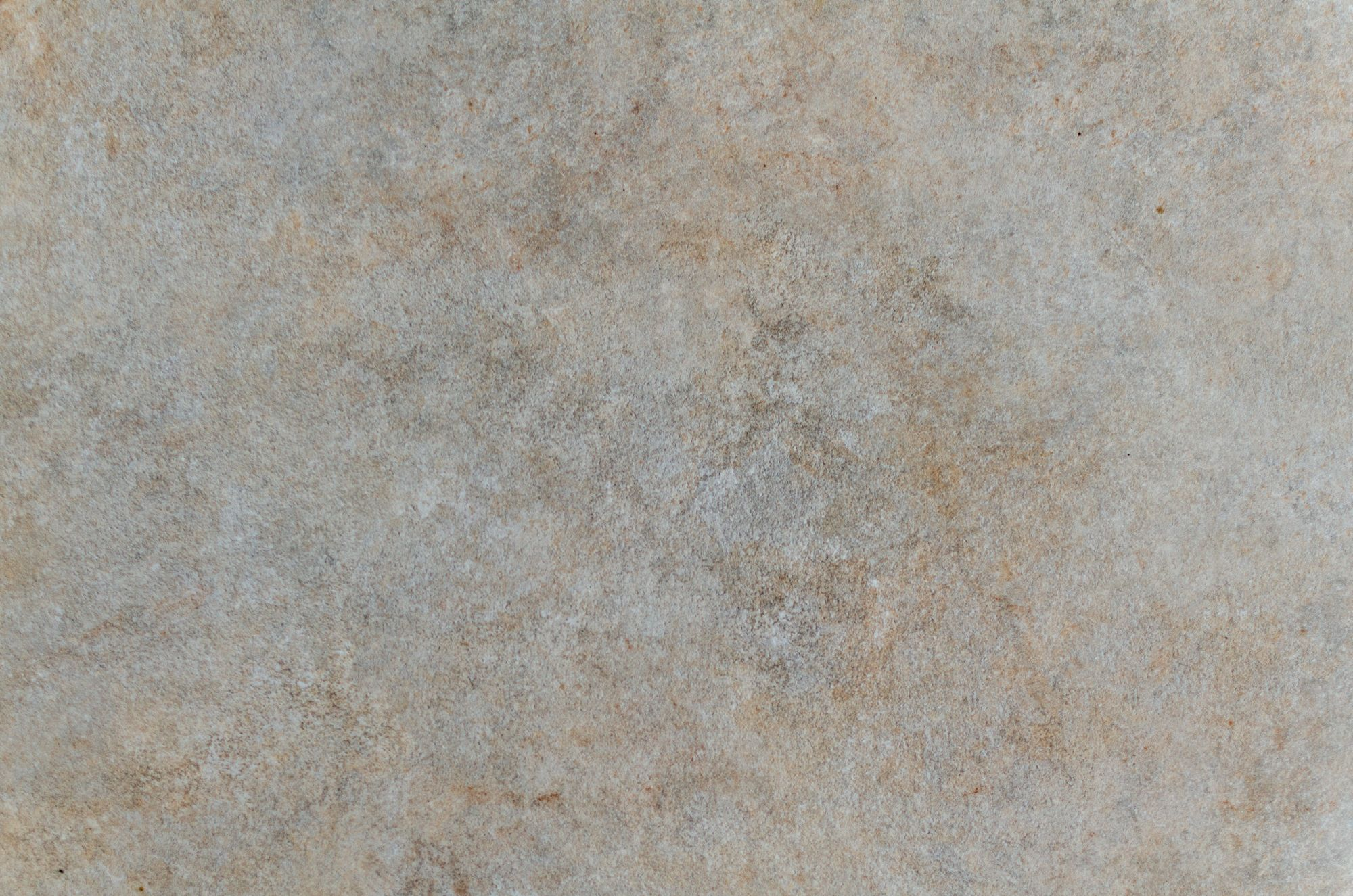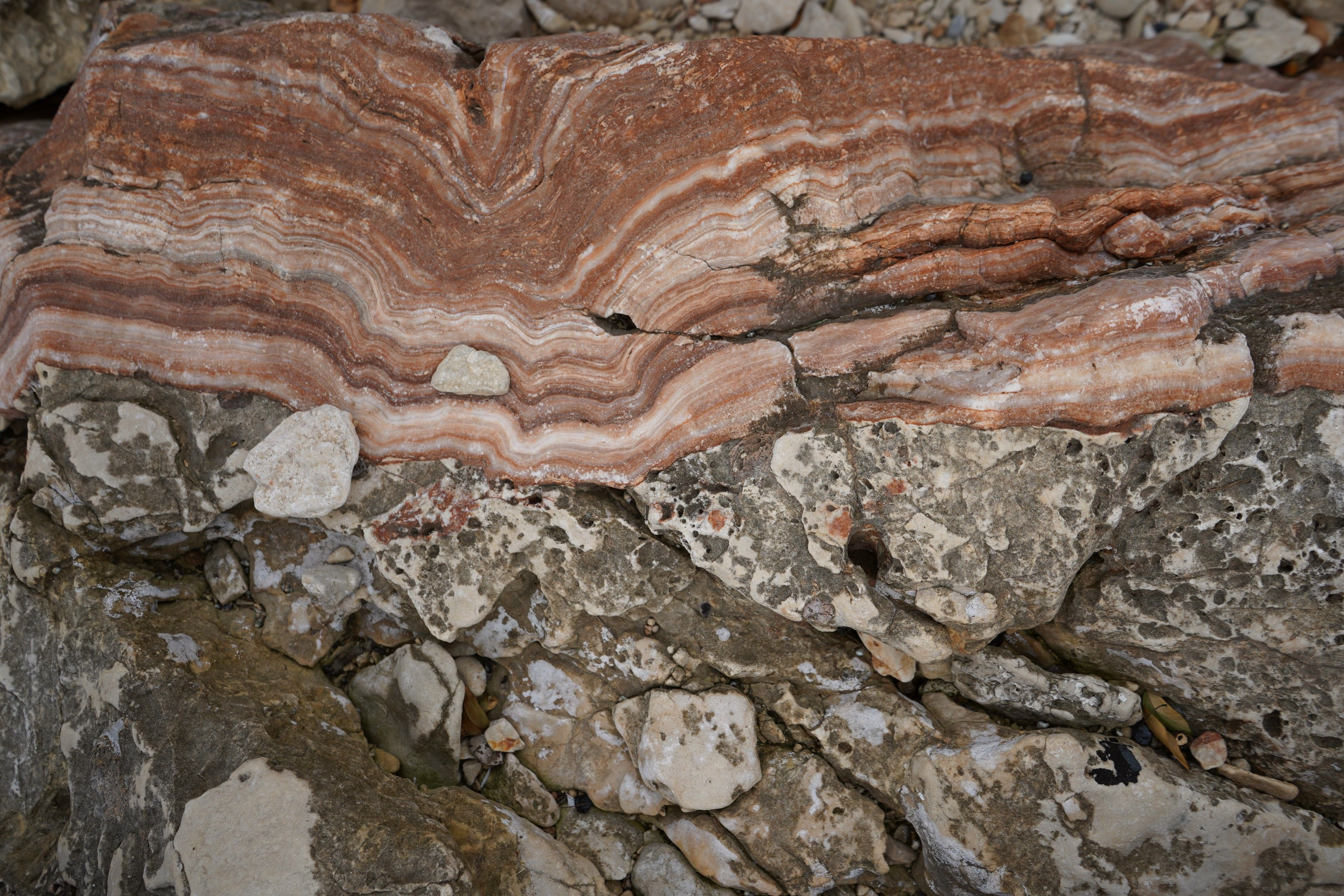# What math do you need for abstract algebra?

What Math Do You Need for Abstract Algebra?

# What Math Do You Need for Abstract Algebra?If you’re interested in diving into the fascinating and complex world of abstract algebra, the first thing you need to know is that it’s not like your typical algebra course. Abstract algebra is a branch of mathematics that goes beyond manipulating numbers and equations to explore the more abstract structures and concepts underlying algebraic systems. It’s a challenging subject that requires a strong foundation in math, but the good news is that the prerequisites for self-studying abstract algebra are surprisingly low. In this guide, we’ll cover everything you need to know about the math prerequisites for studying abstract algebra.

## What Are the Prerequisites for Studying Abstract Algebra?

As mentioned earlier, the requirements for self-studying abstract algebra are surprisingly low. Here’s what you need to know:

### 1. Pre-calculus Mathematics

Before you dive into abstract algebra, you should be familiar with most pre-calculus mathematics. This includes:

• Functions: how to define them, calculate their values, and graph them.
• Trigonometry: the basic trig functions and their properties, such as sine, cosine, tangent, and their inverses.
• Exponents and logarithms: how to manipulate and simplify expressions involving exponents and logarithms.
• Matrices: how to perform basic operations on matrices, such as addition, subtraction, multiplication, and inverses.
• Basic concepts in calculus: limits, derivatives, and integrals.

If you have taken a pre-calculus or calculus course, you should have already covered these topics. If not, it’s recommended that you brush up on your pre-calculus math skills before diving into abstract algebra.### 2. Understanding Proofs

One of the key differences between abstract algebra and other types of algebra is that abstract algebra focuses heavily on proofs. A proof is a logical argument that demonstrates the truth of a mathematical statement. In other words, it’s a way of showing that something is mathematically true, rather than just taking it on faith. So, before you can study abstract algebra, you need to have a basic understanding of proofs and how they work.

If you’re not familiar with proofs, there are many online resources that can help you get started. Some recommended websites include:

## FAQs

### Q: Can you study abstract algebra without a background in calculus?

A: While a strong background in calculus is helpful, it’s not strictly necessary for studying abstract algebra. However, you will need to be comfortable with some basic calculus concepts, such as limits and derivatives, in order to fully understand some of the more advanced abstract algebra concepts.

### Q: Do you need to be a genius at math to study abstract algebra?

A: No, you don’t need to be a genius at math to study abstract algebra. However, you do need to have a strong foundation in math, a willingness to learn, and a lot of hard work and dedication.### Q: How much time should I dedicate to learning abstract algebra?

A: The amount of time you need to dedicate to learning abstract algebra depends on your current level of math knowledge and your learning style. Some people may be able to pick up abstract algebra quickly, while others may need to spend more time on each concept. It’s recommended that you plan to spend at least a few hours a week studying abstract algebra in order to make steady progress.